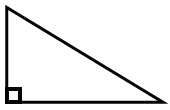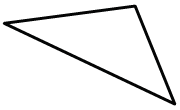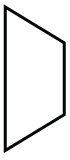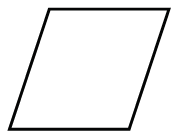### Home > CC2MN > Chapter 4 > Lesson 4.3.1 > Problem4-82

4-82.

For each shape drawn below, choose one of the names on the list above them that best describes that shape. Be as specific as you can. If you do not remember what one of the shape names means, you may look in the glossary of the eBook for more information.

right triangle

scalene triangle

obtuse triangle

equilateral triangle

parallelogram

rectangle

rhombus

trapezoid

acute triangle

1.This triangle has acute angles, so you might think it is an acute triangle. However, it is possible to be more specific, since there is a right angle.

Right or scalene triangle; there is a right angle and all of the sides are different lengths.

1.Is there an angle greater than $90°$? If so, it could be called an obtuse triangle.

1.Certainly this is a quadrilateral, but can you be more specific?

Trapezoid; there are two parallel sides with two non-parallel sides.

1.There are two pairs of parallel sides, and all sides are equal length, meaning you can be more specific than ''parallelogram.''